• +91 9971497814
• info@interviewmaterial.com

# RD Chapter 6- Factorisation of Polynomials Ex-6.1 Interview Questions Answers

### Related Subjects

Question 1 : Which of the following expressions are polynomials in one variable and which are not? State reasons for your answer: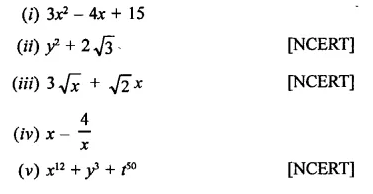(i) 3x2 –4x + 15,
(ii) y2 + 2
√3 arepolynomial is one variable. Others are not polynomial or polynomials in onevariable.

Question 2 : Write the coefficient of x2 in each of the following: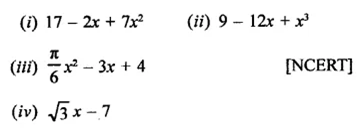Answer 2 : Coefficient of x2,
in (i) is 7
in (ii) is 0 as there is no term of x2 i.e. 0 x2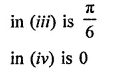Question 3 :
Write the degrees of each of the following polynomials:
(i) 7x3 + 4x2 – 3x + 12
(ii) 12 – x + 2x3
(iii) 5y –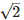(iv) 7
(v) 0

(i)Degree of the polynomial 7x3 + 4x2 – 3x + 12 is3
(ii) Degree of the polynomial 12 – x + 2x3 is 3
(iii) Degree of the polynomial 5y –is 1

(iv) Degree of the polynomial 7 is 0
(v) Degree of the polynomial 0 is 0 undefined.

Question 4 :
(i) x + x2 + 4
(ii) 3x – 2
(iii) 2x + x2 [NCERT]
(iv) 3y
(v) t2 + 1
(v) 7t4 + 4t3 + 3t – 2

(i)  x + x2 + 4 It is a quadraticpolynomial.
(ii) 3x – 2 : It is a linear polynomial.
(iii) 2x + x2: It is a quadratic polynomial.
(iv) 3y It is a linear polynomial.
(v) t2+ 1 It is a quadratic polynomial.
(vi) 7t4 + 4t3 + 3t – 2 It is a biquadraticpolynomial.

Question 5 : Classify the following polynomials as polynomials inone-variable, two-variables etc.
(i) x2-xy +7y2
(ii) x2 – 2tx + 7t2 – x + t
(iii) t3 -3t2 + 4t-5
(iv) xy + yz + zx

(i) x2 – xy + 7y2: It is a polynomialin two j variables x, y.
(ii) x2 – 2tx + 7t2 – x + t: It is a polynomialin two variables in x, t.
(iii) t3 – 3t2 + 4t – 5 : It is a polynomial inone variable in t.
(iv) xy +yz + zx : It is a polynomial in 3 variables in x, y and

Question 6 : Identify polynomials in the following: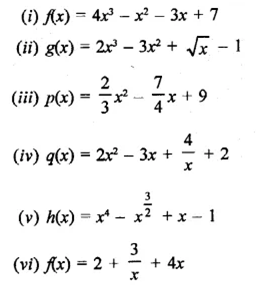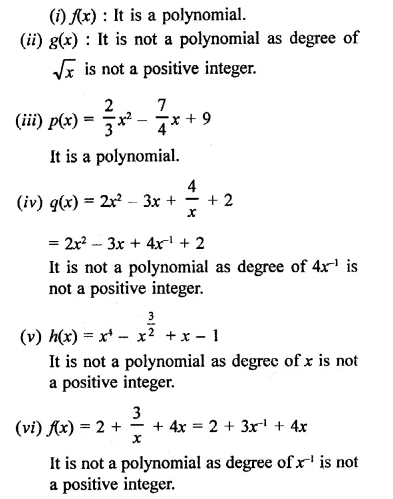Question 7 : Identify constant, linear, quadratic and cubic polynomials from the following polynomials: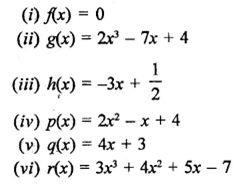(i)f(x) = 0 : It is a constant polynomial as it has no variable.
(ii) g(x) = 2x3 – 7x + 4 : It is a cubic polynomial.
(iii) h(x) = -3x +
12 :It is a linear polynomial.
(iv) p(x) = 2x2 – x + 4 : It is a quadratic polynomial.
(v) q(x) = 4x + 3 : It is linear polynomial.
(vi) r(x) = 3x3 + 4x2 + 5x – 7 : It is acubic polynomial.

Question 8 : Give one example each of a binomial of degree 35 and of a monomial of degree 100.   [NCERT]

Example of a binomial of degree 35 = 9x35 + 16
Example of a monomial of degree 100 = 2y100

Todays Deals### RD Chapter 6- Factorisation of Polynomials Ex-6.1 Contributorskrishan

Name:
Email:

# Latest News# 9000 interview questions in different categories